Trigonometry : Graphing Tangent and Cotangent

Example Questions

Example Question #64 : Trigonometric Functions And Graphs

Which of the following best describes where the asymptotes are located on a tangent graph?

Angle measures where the cosine is 0, such as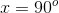Angle measures where the sine and cosine are equal, such as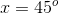.

Angle measures where the sine is 0, such as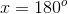.

Angle measures where the tangent cannot be calculated.

Angle measures where the cosine is 0, such asExplanation:

In trigonometry,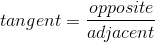.

It may also be thought of as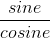.

This is because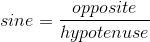and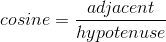, so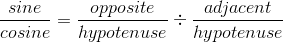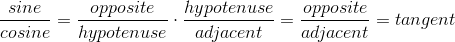This means that whenever cosine is 0, tangent is undefined, because it would be evaluated by dividing by 0.

Example Question #61 : Trigonometric Functions And Graphs

Which of the following is not a solution to the following equation?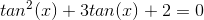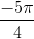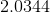...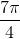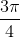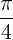Explanation:

We can factor the original expression as follows: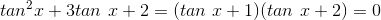So from this equation we conclude either that: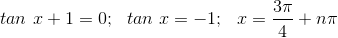or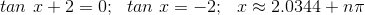So any number that is not some integer multiple ofaway from these two solutions is not a solution to the original equation.

The only such choice is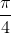, which is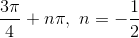; n is not an integer, therefore it is not a solution.

Example Question #1 : Graphing Tangent And Cotangent

The following is a graph of which function?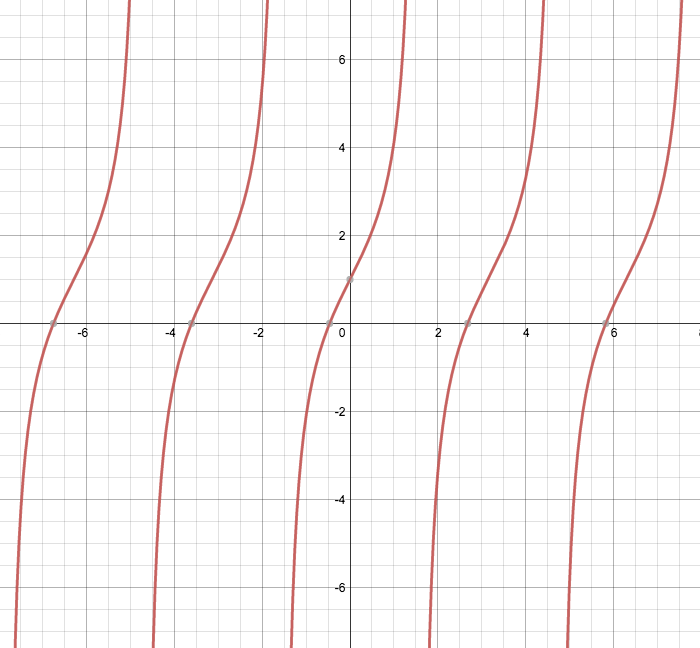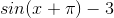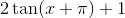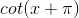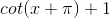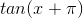Explanation:

The graph looks to have infinite range, but multiple vertical asymptotes. That means we can limit our choices to tangent and cotangent graphs.

Furthermore, we observe that the graph starts at the bottom and increases from left to right, consistent with tangent graphs. So we narrow our focus to the choices involving tangents.

To decide between the remaining two graphs, observe that y-intercept (where x=0) of our graph is (0,1).

Nowevaluated atis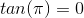, which means that we need a vertical shift ofunit.

Hence the best choice is: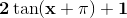All Trigonometry Resources